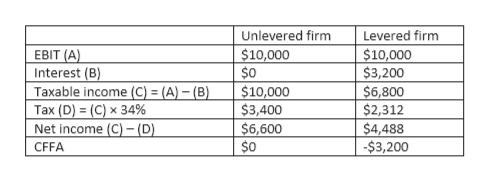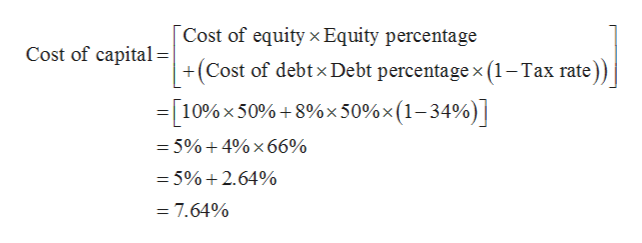# Assuming that there is an unlevered firm and a levered firm. The basic information is given by the following table. Table1: Information of the firms Unlevered firmLevered firmEBIT1000010000Interest03200Taxable income  Tax (tax rate: 34%)  Net income  CFFA   Assuming that cost of debt =8%; unlevered cost of capital =10%; systematic risk of the asset is 1.5Fill in the blanksWhat is the present value of the tax shield?

Question
129 views

Assuming that there is an unlevered firm and a levered firm. The basic information is given by the following table.

Table1: Information of the firms

 Unlevered firm Levered firm EBIT 10000 10000 Interest 0 3200 Taxable income Tax (tax rate: 34%) Net income CFFA

Assuming that cost of debt =8%; unlevered cost of capital =10%; systematic risk of the asset is 1.5

• Fill in the blanks
• What is the present value of the tax shield?
check_circle

star
star
star
star
star
1 Rating
Step 1

The required values are mentioned in below table:help_outlineImage TranscriptioncloseUnlevered firm Levered firm \$10,000 \$0 EBIT (A) \$10,000 \$3,200 Interest (B) Taxable income (C) = (A) - (B) Tax (D) (C) x 34% Net income (C)-(D). \$10,000 \$3,400 \$6,600 \$0 \$6,800 \$2,312 \$4,488 -\$3,200 CFFA fullscreen
Step 2

Unlevered firm will have zero tax shield as no interest on debt is paid.

In order to calculate the present value of tax shield, cost of capital should be known. Assume the weights for equity and debt is 50:50.

The cost of capital is calculated below:help_outlineImage TranscriptioncloseCost of equity x Equity percentage +(Cost of debtx Debt percentage x (1-Tax rate) Cost of capital =[10%x 50%+8%x 50% x (1-34%) = 5%+ 4% x 66% = 5%+2.64% = 7.64% fullscreen
Step 3

The present value of tax shiel...

### Want to see the full answer?

See Solution

#### Want to see this answer and more?

Solutions are written by subject experts who are available 24/7. Questions are typically answered within 1 hour.*

See Solution
*Response times may vary by subject and question.
Tagged in